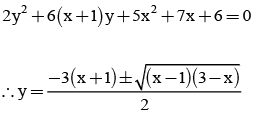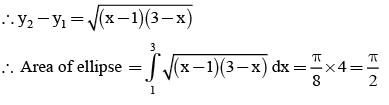Courses

# Test: Area Under Curve With Solution (Competition Level)

## 30 Questions MCQ Test Mathematics (Maths) Class 12 | Test: Area Under Curve With Solution (Competition Level)

Description
This mock test of Test: Area Under Curve With Solution (Competition Level) for JEE helps you for every JEE entrance exam. This contains 30 Multiple Choice Questions for JEE Test: Area Under Curve With Solution (Competition Level) (mcq) to study with solutions a complete question bank. The solved questions answers in this Test: Area Under Curve With Solution (Competition Level) quiz give you a good mix of easy questions and tough questions. JEE students definitely take this Test: Area Under Curve With Solution (Competition Level) exercise for a better result in the exam. You can find other Test: Area Under Curve With Solution (Competition Level) extra questions, long questions & short questions for JEE on EduRev as well by searching above.
QUESTION: 1

### The area bounded by [x] +[y] = 8 such that x, y > 0 is .... sq. units Where [.] is G.I.F.

Solution:

Area of 9 unit squares = 9 sq.units

QUESTION: 2

### Let f : [0, ∞)→ R be a continuous and strictly increasing function such that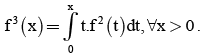The area enclosed by y = f (x), the x-axis and the ordinate at x = 3, is

Solution:

Given, f3(x) = ∫(0 to x)tf(t)dt
Differentiating throughout w.r.t x,
3f2(x) f′(x) = xf2(x)
⇒ f′(x)= x/3
​So, f(x)=∫(0 to x) x/3 dx = (x2)/6
Area = ∫f(x)dx=∫(0 to 3) (x2)/6 dx
= {(x3)/18}(0 to 3)
= 3/2

QUESTION: 3

### The area bounded by x = x1, y = y1 and y = -(x + 1)2 where x1, y1 are the values of x, y satisfying the equation sin-1x + sin-1y = -π will be, (nearer to origin)

Solution: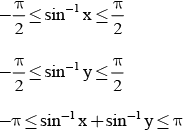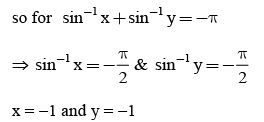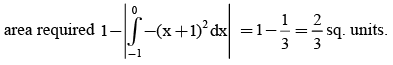QUESTION: 4

Area bounded between the curves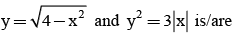Solution: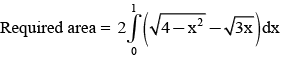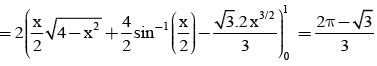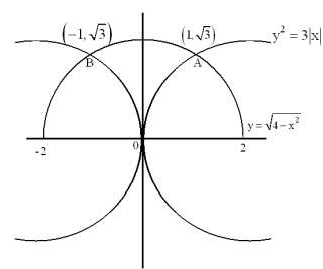QUESTION: 5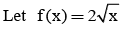and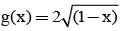be two functions and let f1(x) = max {f(t), 0 < t < x, 0 < x <} and g1(x) = min {g(t), 0 < t < x, 0 < x < 1}. Then the area bounded by f1(x) < 0, g1(x) < 0 and x-axis is

Solution:
QUESTION: 6

The values of the parameter a(a > 1) for which the area of the figure bounded by the pair of straight lines y2 – 3y + 2 = 0 and the curves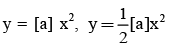is greatest is.
(Here [.] denotes the greatest integer function).

Solution:

The curves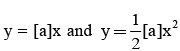represent parabolas which are symmetric about yaxis. The equation y2 – 3y + 2 = 0 gives a pair of straight lines y = 1, y = 2 which are parallel to x-axis. The shaded region in figure determines the area bounded by the two parabolas and two lines. Let us slice this region into horizontal strips. For the approximating rectangle shown in figure. We have Length = x2 – x1, width = Δy, Area = (x2 - x1)dy
AT it can move vertically y = 1 and y = 2. So, Required area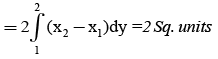QUESTION: 7

Area of region bounded by x2 + y2 < 4 and (|x| + |y|) < 2 is ____ square units.

Solution: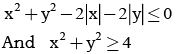Using circles representation, required area = 8 sq. units

QUESTION: 8

The area of a circle is A1 and the area of a regular pentagon inscribed in the circle is A2 .Then A1 : A2 is

Solution: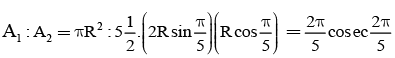QUESTION: 9

The area bounded by the curve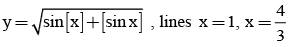and x-axis is

Solution: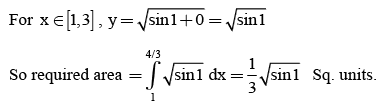QUESTION: 10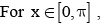the area of the region bounded by y = x and y = x+ sin x is

Solution:QUESTION: 11

The area bounded by the curves y = x2, y = [x+1], x < 1 and the y - axis, where[.] denotes the greatest integer not exceeding x, is

Solution: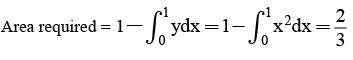QUESTION: 12

The area of the smaller region in which the curve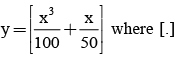denotes the greatest integer function, divides the circle (x – 2)2 + (y + 1)2 = 4, is equal to

Solution:

Circle has (2, -1) as its centre and radius of this circle is 2.
Thus if P(x, y) be any point on it, then x ∈ [0, 4].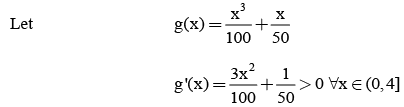The g(x) is increasing in [0, 4]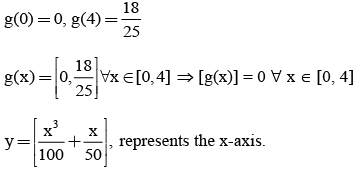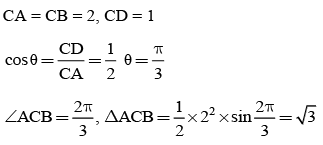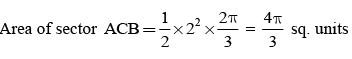QUESTION: 13

The area bounded by the curves y = 2- |x -1| , y = sin x ; x = 0 and x =2 is

Solution: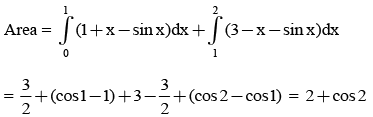QUESTION: 14

A curve passes through the point (0,1)and has the property that the slope of the curve at every point P is twice the y–coordinate of P . If the area bounded by the curve, the axes of coordinates and the line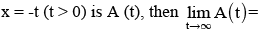Solution: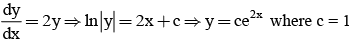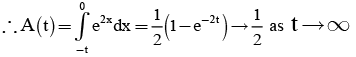QUESTION: 15

The area bounded by the curve (y – arc sinx)2 = x – x2, is

Solution: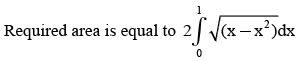QUESTION: 16

The area of the region enclosed by the curve 5x2 + 6xy + 2y2 + 7x + 6y + 6 = 0 is

Solution:

Comparing the given equation with the general equation of second degree i.e.
ax2 + 2hxy + by2 + 2gx + 2fy + c = 0,
We have, abc + 2fgh – af2 – bg2 – ch2 = 60 – 45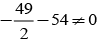And h2 – ab = 9 – 10 < 0.
So, the given equation represents an ellipse.
Rewriting the given equation as a quadratic in y, we obtain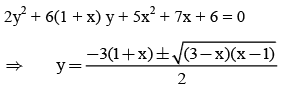Clearly, the values of y are real for x ∈ [1, 3]
When x = 1, we get y = -3 and x = 3 ⇒ y = -6.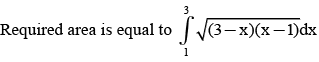QUESTION: 17

Let f(x) be a continuous function such that the area bounded by the curve y = f(x), the xaxis and the two ordinates x = 0 and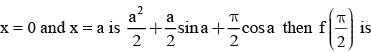Solution: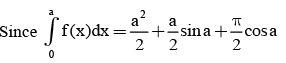Differentiating both sides w.r.t. a, we get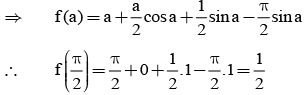QUESTION: 18

The area enclosed between the curves y = sin4x cos3x, y = sin2xcos3x between x = 0 and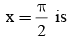Solution:

Required area is equal to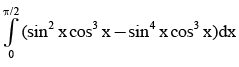QUESTION: 19

The area of the region bounded by the curves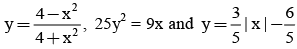which contains (1, 0) point in its interior is

Solution: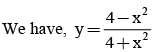We observe that all powers of x in the above equation are even, so it is symmetric about y-axis. The curve intersects y-axis at (0, 1). Also,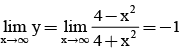Therefore, as x → ∞, y → -1 i.e. y = -1 is an asymptote to the curve.This means that x is imaginary for y > 1 or y < -1 i.e. the curve lies between y = -1 and y = 1. At (0, 1) the tangent to the curve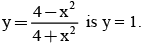QUESTION: 20

Area bounded by the curves y = ex , y= loge x and the lines x = 0, y = 0, y = 1 is

Solution: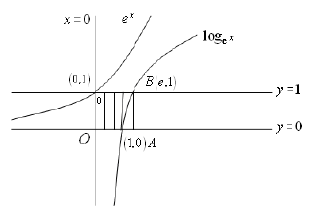Area = Area of  rectangle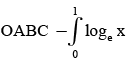QUESTION: 21

A circular arc of radius ‘1’ subtends an angle of ‘x’ radians,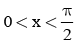as shown in the figure. The point ‘R’ is the point of intersection of the two tangent lines at P & Q. Let T(x) be the area of triangle PQR and S(x) be area of the shaded region. Then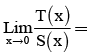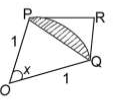Solution: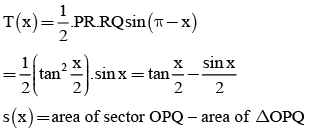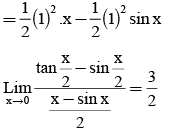QUESTION: 22

A point P moves inside a triangle formed by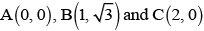such that  min {PA, PB, PC} = 1. The area formed by the curve traced by P is ....... sq. units

Solution: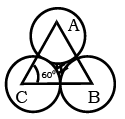Re quired area = Area of equilateral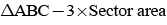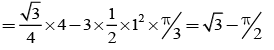QUESTION: 23

Area of the triangle formed by the tangent and normal at (1, 1) on the curve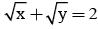and the y-axis is

Solution:

Find equation of the tangent and normal and then put x=0 to evaluate vertices of triangle.
Then find area of triangle .

QUESTION: 24

Area bounded by the curve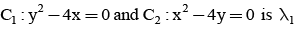and area bounded by latus rectum of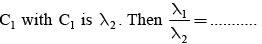Solution:

Area bounded by C1 & C2 is λ1 = 16/3 sq.units
λ2 = Area bounded by C1 & its latus rectum = 8/3 sq.units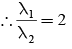QUESTION: 25

The area of the part of circle x2 + y2 - 2x - 4y -1 = 0 above 2x - y = 0 is ...... sq. units

Solution:

Required area = Area of semi-circle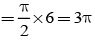Since given line is diameter of circle

QUESTION: 26

The area bounded by the curves y = |x| – 1 and y – |x| 1is

Solution:
QUESTION: 27

Area of closed curve 3(x -1)2 + 4(y2 - 3) = 0 is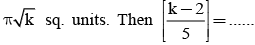where [.] is G.I.F

Solution: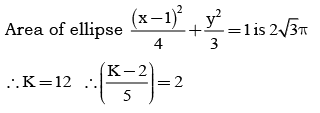QUESTION: 28

Let f(x) = x + sin x. The area bounded by y = f-1 (x), y = x, x ∈ [0, π] is

Solution:

The curves given by y = x + sin x and y = f-1(x) are images of each other in the line y = x.
Hence required area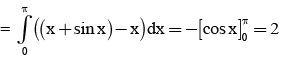QUESTION: 29

The area of the region bounded by the curves |x + y| < 2, |x – y| < 2 and 2x2 + 6y2 > 3 is

Solution:

2x2 + 6y2 > 3. . . . . . (1) area of ellipse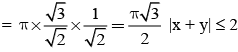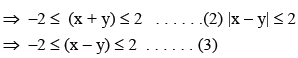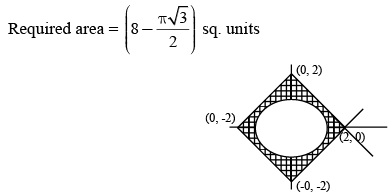QUESTION: 30

Area enclosed by the closed curve 5x2 + 6xy + 2y2 + 7x + 6y + 6 = 0 is

Solution: